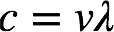# Problem: A carbon–oxygen double bond in a certain organic molecule absorbs radiation that has a frequency of 6.0 x 1013 s-1. What is the wavelength of this radiation?

###### FREE Expert Solution

Recall: The relationship between frequency ν and wavelength λ is:where c = speed of light, 3.00 × 108 m • s–1. We're given radiation with a frequency = 6.0 x 1013 s–1.

99% (281 ratings)###### Problem Details

A carbon–oxygen double bond in a certain organic molecule absorbs radiation that has a frequency of 6.0 x 1013 s-1. What is the wavelength of this radiation?